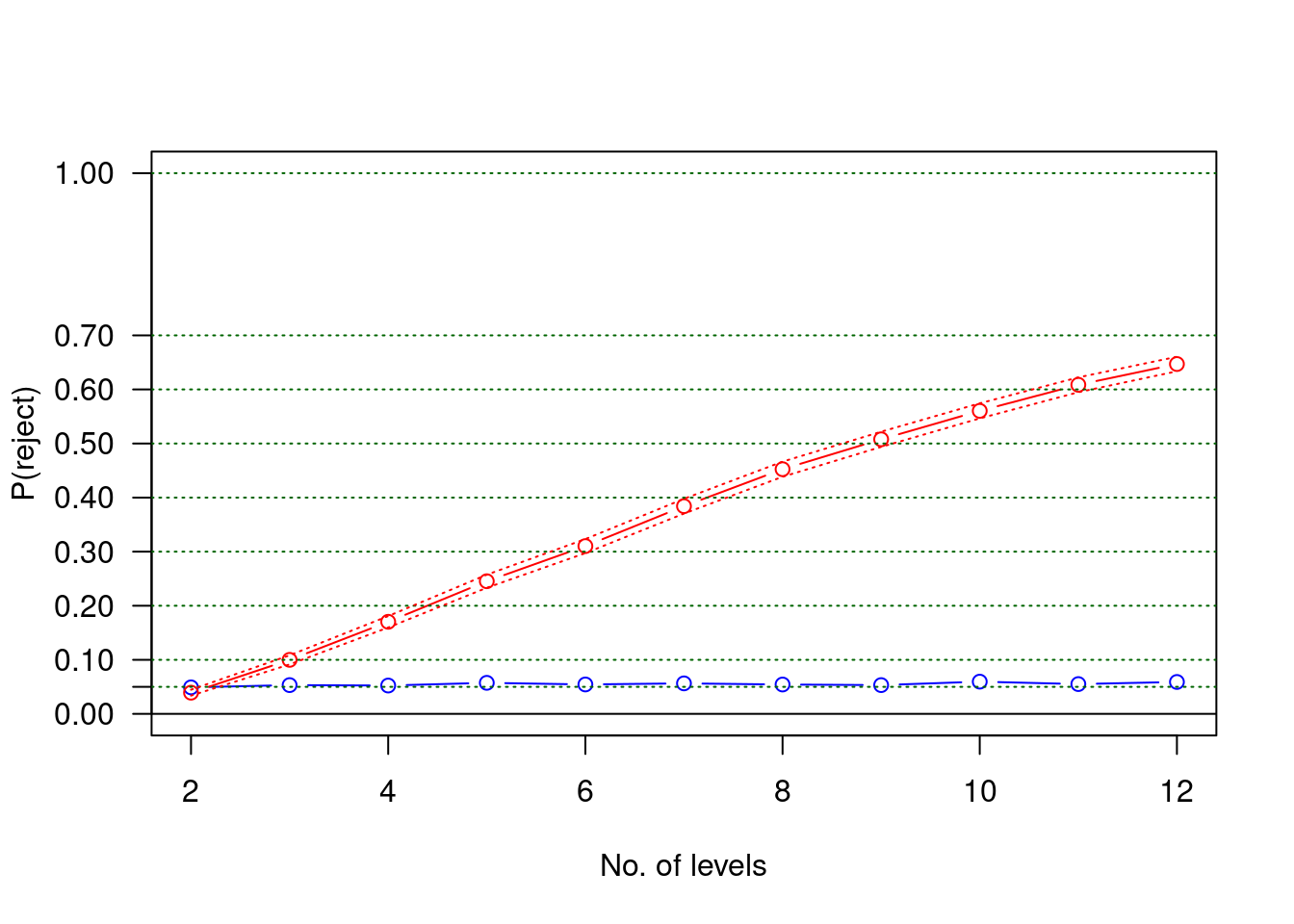# 1 The problem

When I run a logistic regression on infant mortality with a categorical covariate, say season, with four categories, (‘spring,’ ‘summer,’ ‘fall,’ ‘winter’), with the following data (400 births),

``````  deaths births season
1     50    100 spring
2     44    100 summer
3     58    100   fall
4     41    100 winter``````

I get this output from R, with winter as reference category:

``````             Estimate Std. Error z value Pr(>|z|)
(Intercept)   -0.3640     0.2033 -1.7901   0.0734
seasonspring   0.3640     0.2852  1.2762   0.2019
seasonsummer   0.1228     0.2862  0.4290   0.6679
seasonfall     0.6867     0.2870  2.3925   0.0167``````

Hmm, interesting, the smallest p-value is around 1-2%. But I think I want spring as reference category when I present it.

``````             Estimate Std. Error z value Pr(>|z|)
(Intercept)    0.0000     0.2000  0.0000   1.0000
seasonwinter  -0.3640     0.2852 -1.2762   0.2019
seasonsummer  -0.2412     0.2839 -0.8495   0.3956
seasonfall     0.3228     0.2847  1.1338   0.2569``````

Oops, what happened to my small p-value?

## 1.1 The cause

The answer is that in each case we only reported three contrasts (pairwise comparisons), but there is a total of six contrasts. In the second case we are missing the contrast with the largest difference, ‘fall’ vs. ‘winter’.

# 2 The solution

Our conclusions shouldn’t depend on how we code our data, or what reference category we choose. We need the likelihood ratio test (LRT):

``````Single term deletions

Model:
cbind(deaths, births - deaths) ~ season
Df Deviance    AIC    LRT Pr(>Chi)
<none>      0.0000 28.174
season  3   6.7821 28.956 6.7821  0.07918``````

This result is invariant under reparametrization. If we a priori wanted to compare winter and fall, we could eliminate cases with season equal to spring or summer and get:

``````            Estimate Std. Error z value Pr(>|z|)
(Intercept)  -0.3640     0.2033 -1.7901   0.0734
seasonfall    0.6867     0.2870  2.3925   0.0167``````
``````Single term deletions

Model:
cbind(deaths, births - deaths) ~ season
Df Deviance    AIC    LRT Pr(>Chi)
<none>      0.0000 14.065
season  1   5.8088 17.874 5.8088  0.01595``````

As you can see, this reduced case gives the same “Wald” p- value (= 0.0167) as the original case, while the “LRT” p-value is sligthly smaller, 0.0159.

## 2.2 Back to the main track: A simulation study

Back to the “real business.” I simulated situations with a categorical variable with different numbers of levels. The null situation is under investigation, and the real significance level will be compared to the nominal, which I choose to be 5%. For each No. of levels, 2, …, 12, ten thousand replicates were made of an experiment with 100 births at each level and the probability of death constantly equal to 10% (a reasonable number in nineteenth century Västerbotten). I have tried other combinations of sample sizes and true death probability, but the general conclusion does not change.

The number of levels is increasing from two to twelve, and for each I estimate the probability of finding a “significant” contrast. Since everything is done under the null hypothesis of no differences, we hope this probability to be below 5%. This is what happens:The blue line shows the overall (LRT) rejection probability as a function of the number of levels, and it stays almost constant at 0.05, as it should. The red line (with uniform 95% confidence limits) shows the probability of at least one “significant” contrast as a function of the number of levels, and it is OK for two levels, but then it quickly goes wild. We do no want to use a rule that has a 60% probability of Type 1 error (11 levels), do we?

# 3 Conclusion

The correct procedure is to first look at the overall (LRT) p-value, and only if that is small enough, go on and examine contrasts, but concentrate on the effect sizes rather than (exaggerated) p-values. Formal procedures for doing this have been around for almost a century, so study the old masters .

The (simple) Holm procedure can be described as follows: For n null hypotheses, calculate the traditional p-values and order them in increasing order. Then start with the smallest: if it is larger than 0.05/n, forget the whole thing. If it is smaller, reject the corresponding hypothesis and go on to the next ordered p-value and compare it with 0.05/(n-1): reject if smaller, otherwise forget this and the remaining hypotheses. And so on, until a p-value is too large. Note that for each rejection, the limit is made slightly larger. This is the improvement (more power) over the classical Bonferroni procedure that Holm (1979) introduced and extended to subset selection procedures (Sture Holm was my thesis supervisor 1978-79).

Finally some bad(?) news: This problem with multiple testing applies to all situations where more than one p-value is wanted, not only the case with one categorical covariate with many levels. There are two practical approaches to take: (i) Follow Holm’s rule as desribed above, or (ii) Regard p-values as ordinary statistics (they are), and give no probability interpretations to them, essentially, skip the stars. Do not test hypotheses about contrasts, satisfy yourself with the LRT tests. Ignoring these rules leads sooner or later to the territory where scientific fraud lingers. Most important is to have a research protocol, written before looking at data, stating exactly which hypotheses you want to test.

And, by all means, do not forget the subject matter significance.